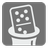Magic Domino
Simple+
English RU

When Nikola can not find opponent for a game of Dominoes, he spreads them out for a game of Magic Domino Solitaire. The goal of solitaire is to build a Magic square with Double Six domino tiles.

A magic square is an arrangement of distinct integers, in a square grid, where the numbers in each row, and in each column, and the numbers in the forward and backward main diagonals, all add up to the same number.
Domino tiles contain two numbers from 0 (empty) to 6. Tiles are unordered and 1-6 is the same as 6-1. The double-six set contains 28 unique tiles - all combinations of number pairs.You are given a size for the magic square and a number. You should build a magic square to the given size so that the sum of the horizontal and vertical diagonals equal the given number. You can place domino tiles from double-six set only vertically and they should be unique.

Here is example for size = 4 and number = 5:The result magic square should be represented as a list/tuple of lists/tuples with integers.

Input: Two arguments. A magic square size and a number as integers

Output: The magic square as a list/tuple of lists/tuples with integers.

Example:

```checkio(4, 5) == ((0, 0, 2, 3),
(0, 4, 1, 0),
(4, 0, 0, 1),
(1, 1, 2, 1))
```

How it is used: This is a constraint satisfaction problem. It's used not only for solving games of solitaire, but also for planning and resource allocation in city planning, construction and just about anything else.

Precondition:
size in (4, 6)
All input test cases are solvable.